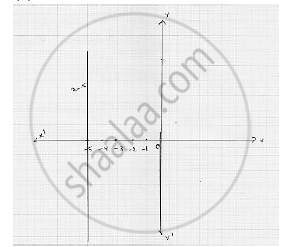# Solve the Equation 3x + 2 = X − 8, and Represent the Solution on the Cartesian Plane . - Mathematics

Solve the equation 3x + 2 = x − 8, and represent the solution on the
Cartesian plane .

#### SolutionOn Cartesian plane, equation represents all points on y - axis  for which  x = 5

Concept: Equations of Lines Parallel to the X-axis and Y-axis
Is there an error in this question or solution?

#### APPEARS IN

RD Sharma Mathematics for Class 9
Chapter 7 Linear Equations in Two Variables
Exercise 7.4 | Q 3.2

Share